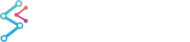﻿ Elliot Wave Drawing Tool | WPF Chart DocumentationSciChart WPF 2D Charts > The Trading Drawing Tools API > Elliot Wave Drawing Tool
Elliot Wave Drawing Tool

The ElliotWaveAnnotation allows a user to draw a 1-2-3-4-5 wave structure on a price chart. To create this annotation you have to set points at your wave turning-points that will be connected by lines. Those points were limited by BasePointsCount (default value is 6).

Note: The Drawing Tools API in SciChart.Charting.DrawingTools is available in the Enterprise and SDK Editions of SciChart onlyThe ElliotWaveAnnotation is derived from TradingAnnotationBase. This is a CompositeAnnotation, which shares the common properties of TradingAnnotationBase and consists of:

Additional properties specific to ElliotWaveAnnotation include:

• TextStyle - defines the style for the text which is displayed at the turning points

## Declaring an ElliotWaveAnnotation in XAML

To declare an ElliotWaveAnnotation in XAML you have to declare the annotation and then declare its InitialBasePoints collection that is actually collection of ComparablePoint objects. ComparablePoint stores X and Y values that are used for placing annotation point. InitialBasePoints collection is limited by BasePointsCount value. And default value is 6.

Declaring an ElliotWaveAnnotation in XAML
Copy Code
```<!-- XAxis, YAxis, RenderableSeries omitted for brevity -->
<s:SciChartSurface.Annotations>
<!--Declare ElliotWaveAnnotation-->
<s:ElliotWaveAnnotation IsEditable="True">
<!-- Declare InitialBasePoints collection that is used for
creating annotation from XAML.
InitialBasePoints is actually observable collection of
ComparablePoint-s. ComparablePoint stores X and Y point Value.
-->
<s:ElliotWaveAnnotation.InitialBasePoints>
<!-- Placing first point -->
<s:ComparablePoint X="0"  Y="0"/>
<!-- Placing second point -->
<s:ComparablePoint X="2"  Y="4"/>
<!-- Placing third point -->
<s:ComparablePoint X="4"  Y="0"/>
<!-- Placing fourth point -->
<s:ComparablePoint X="6"  Y="-4"/>
<!-- Placing fifth point -->
<s:ComparablePoint X="9"  Y="0"/>
<!-- Placing sixth point -->
<s:ComparablePoint X="11"  Y="-4"/>
</s:ElliotWaveAnnotation.InitialBasePoints>
</s:ElliotWaveAnnotation >
</s:SciChartSurface.Annotations>
```

Please Note that the ElliotWaveAnnotation has limit of 6 base points. As you can see here, you just have to set this 6 points for FibonacciExtensionAnnotation: [X1=0; Y1=0], [X2=2; Y2=4], [X3=4; Y3=0], [X4=6; Y4=-4], [X5=9; Y5=0], [X6=11; Y6=-4] .

## Declaring an ElliotWaveAnnotation in Code-Behind

To add an ElliotWaveAnnotation in code, simply use the following C# code:

Declaring the ElliotWaveAnnotation in code
Copy Code
```// Create a SciChartSurface. XAxis, YAxis omitted for brevity
var sciChartSurface = new SciChartSurface();
// Create a ElliotWaveAnnotation.
var elliotWave = new ElliotWaveAnnotation();
// ElliotWaveAnnotation should be added to
//SciChartSurface.Annotation collection first, to make SetBasePoint() method
//works correctly
// Set first point of ElliotWaveAnnotation
elliotWave.SetBasePoint(0, 0);

// Set second point of ElliotWaveAnnotation
elliotWave.SetBasePoint(2, 4);
// Set third point of ElliotWaveAnnotation
elliotWave.SetBasePoint(4, 0);
// Set fourth point of ElliotWaveAnnotation
elliotWave.SetBasePoint(6, -4);
// Set fifth point of ElliotWaveAnnotation
elliotWave.SetBasePoint(9, 0);
// Set sixth point of ElliotWaveAnnotation
elliotWave.SetBasePoint(11, -4);
```

## Declaring an ElliotWaveAnnotation in MVVM

To add an ElliotWaveAnnotation via MVVM please follow the instructions for adding annotations with MVVM: Declaring Annotations in MVVM with the AnnotationsBinding Markup Extension

To add a ElliotWaveAnnotation via a ViewModel you have to use SciChart markup extension called AnnotationsBinding,

The AnnotationsBinding Markup Extension
Copy Code
```<s:SciChartSurface Annotations="{s:AnnotationsBinding Annotations}">
</s:SciChartSurface>
```

Now you need to create collection for Annotations in ViewModel. Then create and add an ElliotWaveAnnotationViewModel to that collection of Annotations like this:

Declaring the ElliotWaveAnnotation in a ViewModel
Copy Code
```public class MyViewModel : INotifyPropertyChanged
{
public ObservableCollection<IAnnotationViewModel> Annotations { get; }

public void Foo()
{
// Create a ElliotWaveAnnotation.
elliotWave = new ElliotWaveAnnotationViewModel();
// ElliotWaveAnnotation should be added to
//SciChartSurface.Annotation collection first, to make SetBasePoint() method
//works correctly
// Set first point of ElliotWaveAnnotation
elliotWave.SetBasePoint(0, 0);

// Set second point of ElliotWaveAnnotation
elliotWave.SetBasePoint(2, 4);
// Set third point of ElliotWaveAnnotation
elliotWave.SetBasePoint(4, 0);
// Set fourth point of ElliotWaveAnnotation
elliotWave.SetBasePoint(6, -4);
// Set fifth point of ElliotWaveAnnotation
elliotWave.SetBasePoint(9, 0);
// Set sixth point of ElliotWaveAnnotation
elliotWave.SetBasePoint(11, -4);
}
}
```